# Math in Focus Grade 3 Chapter 2 Practice 4 Answer Key Rounding Numbers to Estimate

Go through the Math in Focus Grade 3 Workbook Answer Key Chapter 2 Practice 4 Rounding Numbers to Estimate to finish your assignments.

## Math in Focus Grade 3 Chapter 2 Practice 4 Answer Key Rounding Numbers to Estimate

Mark (✗) each number on the number line.

Question 1.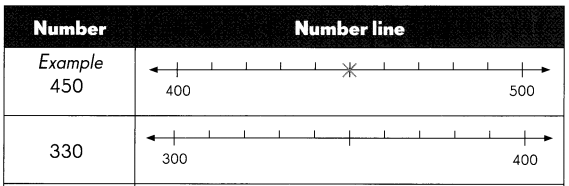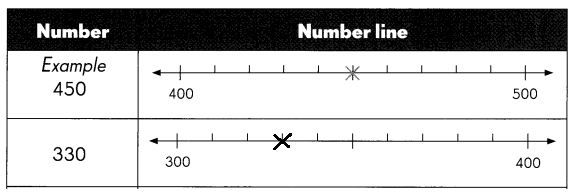Question 2.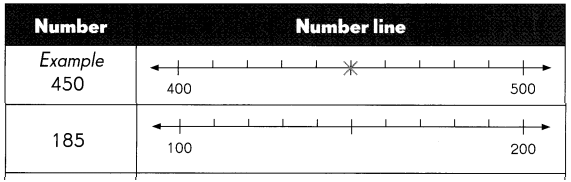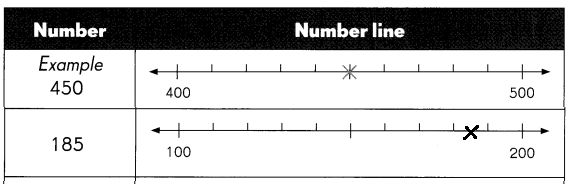Question 3.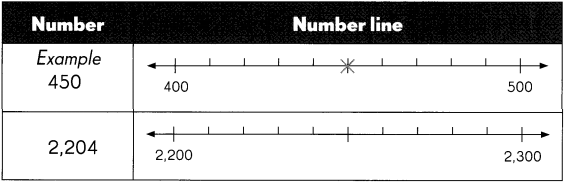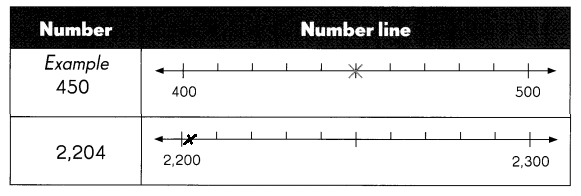Fill in the blanks.

Example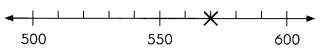570 is nearer to 600 than to 500
570 is 600 when rounded to the nearest hundred.

Question 4.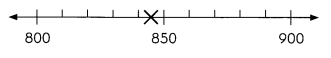845 is nearer to ___ than to ____
845 is ___ when rounded to the nearest hundred.

845 is nearer to 800 than to 900
845 is 800 when rounded to the nearest hundred.

Question 5.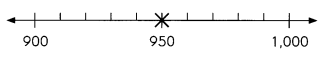950 is nearer to ___ than to ____
950 is ___ when rounded to the nearest hundred.
950 is nearer to 900 or 1000
950 is 900 or 1000 when rounded to the nearest hundred.

Decide whether to find an estimate or an exact answer. Solve.

Example
An organization has about 600 members,
The greatest possible number of members this con be is 649.
The least possible number of members could be 550

Question 6.
Tanya had $900. After buying two of these items, she has$210 left.
a. How much do the two items cost?
Given,
Tanya had $900. She left with$210.

a)
$900 –$210 = $690 She left with$690.

b. Which two items does she buy?

b)
She bought TV and Washing Machine worth $450 and$240.Find the sum or difference. Use rounding to check that each answer is reasonable.

Example
763 + 136 = 899
800 + 100 = 900
So, 763 + 136 is about 900
899 is close to 900, so the answer is reasonable.

Question 7.
238 + 598 = ___
___ + ___ = ___
So, 238 + 598 is about ____
___ is close to ___, so the answer is reasonable.
238 + 598 = 836
200 + 600 = 800
So, 238 + 598 is about 800
836 is close to 800, so the answer is reasonable.

Question 8.
846 – 694 = ____
___ – ___ = ____
So, 846 – 694 is about ___
___ is close to ___, so the answer is reasonable.
846 – 694 = 152
800 – 700 = 100
So, 846 – 694 is about 100
152 is not close to 100, so the answer is not reasonable.

Question 9.
872 – 259 = ___
___ – ___ = ____
So, 872 – 259 is about ___
___ is close to ___, so the answer is reasonable.
872 – 259 = 613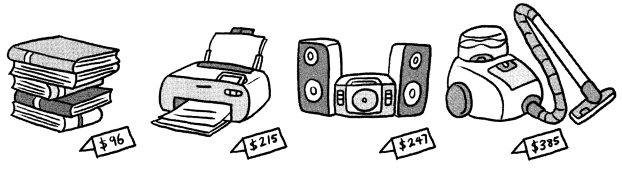Answer: Total cost of the items = $96 +$215 + $247 +$385 + $943 b. Mrs. Ramsey has$980. Does she have enough money to buy all the items?
Answer: Yes, she has enough money to buy all the items. $990 –$943 = \$37.